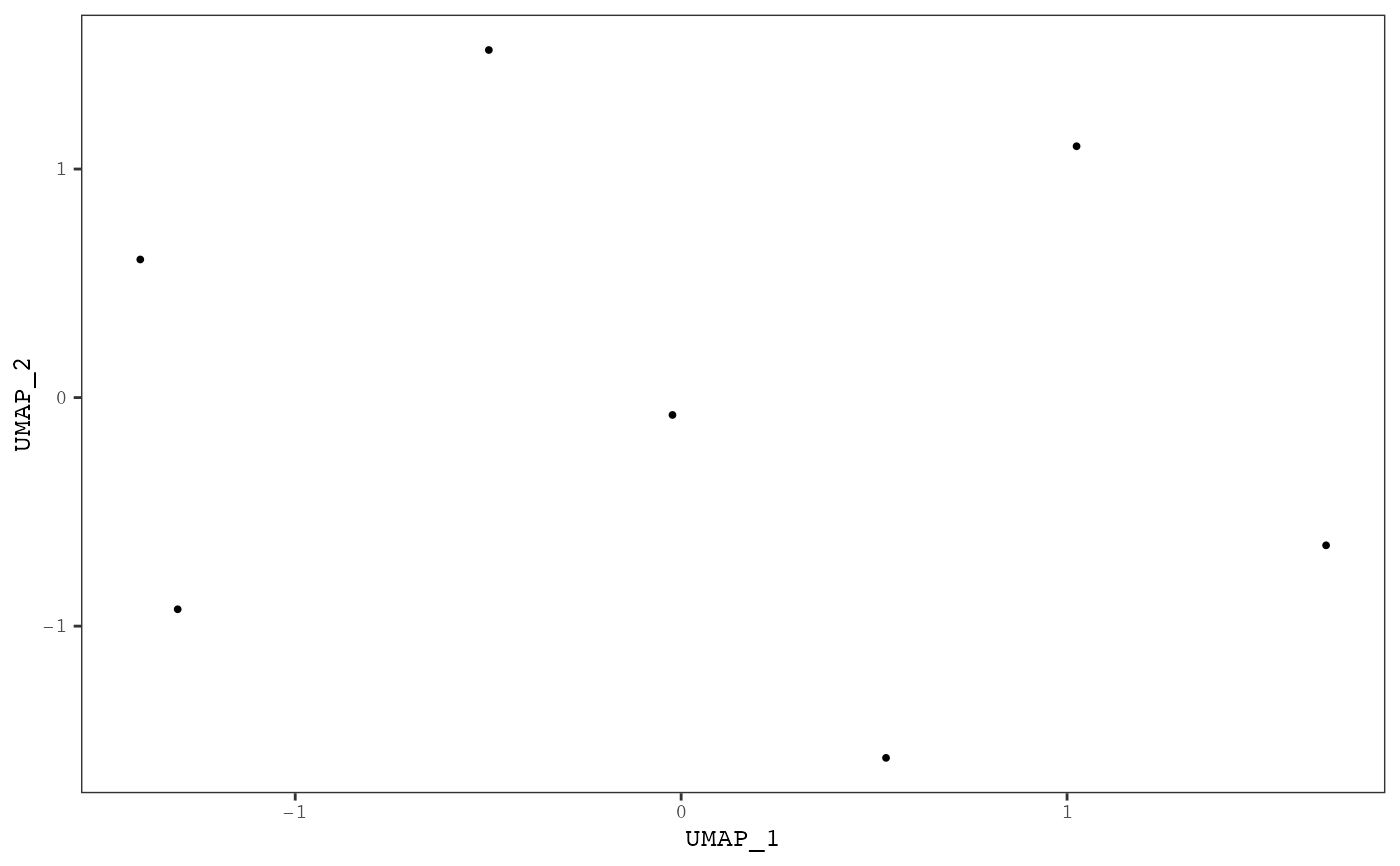Plots samples on a UMAP scatterplot. Samples can be colored by the levels of mutational signatures or by a annotation variable.

plot_umap(
result,
color_by = c("signatures", "annotation", "cluster", "none"),
proportional = TRUE,
annotation = NULL,
point_size = 0.7,
same_scale = TRUE,
annotation_label_size = 3,
annotation_text_box = TRUE,
plotly = FALSE,
clust = NULL,
legend = TRUE,
strip_axes = FALSE
)

Arguments

result A musica_result object generated by a mutational discovery or prediction tool. One of "signatures", "annotation", or "none". If "signatures", then one UMAP scatterplot will be generated for each signature and points will be colored by the level of that signature in each sample. If annotation, a single UMAP will be generated colored by the annotation selected using the parameter annotation. If "none", a single UMAP scatterplot will be generated with no coloring. Default "signature". If TRUE, then the exposures will be normalized to between 0 and 1 by dividing by the total number of counts for each sample. Default TRUE. Sample annotation used to color the points. One used when color_by = "annotation". Default NULL. Scatter plot point size. Default 0.7. If TRUE, then all points will share the same color scale in each signature subplot. If FALSE, then each signature subplot will be colored by a different scale with different maximum values. Only used when color_by = "signature". Setting to FALSE is most useful when the maximum value of various signatures are vastly different from one another. Default TRUE. If TRUE, labels for each group in the annotation variable will be displayed. Only used if codecolor_by = "annotation". This not recommended if the annotation is a continuous variable. The label is plotting using the centriod of each group within the annotation variable. Default FALSE. Size of annotation labels. Only used if codecolor_by = "annotation" and add_annotation_labels = TRUE. Default 3. Place a white box around the annotation labels to improve readability. Only used if codecolor_by = "annotation" and add_annotation_labels = TRUE. Default TRUE. If TRUE, the the plot will be made interactive using plotly. Not used if color_by = "signature" and same_scale = FALSE. Default FALSE. Add cluster labels as annotation Plot legend Remove axes labels for cleaner looking plots

Value

Generates a ggplot or plotly object